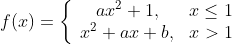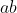# Basic maths-calculus-Q1If f(x) is differentiable at x = 1 then the value ofis _____?asked May 19
reshown May 24

Every differentiable function is countinous. So f(x) is continuous at x=1.
Thus,
left hand limit of f(x) at x= 1 is equal to right hand limit of f(x) at x=1
a*1^2 + 1 = 1^2 + a*1 +b
a+1 = 1+ a+ b

thus b=0
hence ab=0answered May 25 by (180 points)
If f(x) is differentiable at x=1 then

2ax=2x+a

2a=2+a                               at x=1

a=2                                      (i)

If it is differentiable at x=1 then it should be continuous also at x=1

ax^2+1=x^2+ax+b

a+1=1+a+b                         at x=1

2+1=1+2+b                         from (i)

3=3+b

b=0

Therefore the value of ab =0answered May 27 by (112,760 points)